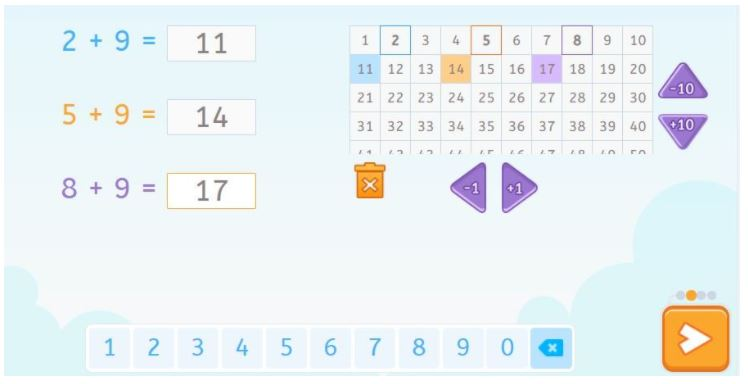Smartick is a fun way to learn math!Dec31

# Addition With and Without Carrying

In today’s post, we are going to learn how to solve addition problems with and without carrying. We will also see some examples of exercises that children complete in Smartick.

But first, let’s review what addition is!

Index

Addition is the act of adding, joining, or putting elements together. When we perform this action we are joining amounts or sets and as a result, there must always be a minimum of two elements.

Humans were performing addition before we even learned to write or had a language. It is believed that many species of animals know how to add small amounts.

## How to Explain Addition to a Primary School Student

Addition is a basic arithmetic operation that we learn at a very young age. Little ones use different strategies to add, such as counting all the elements, counting their fingers, or adding the smaller number to the larger one. We use addition often without realizing it. So if we have to help a child learn to add, it is important that we help them become aware of what they are doing. We can use counters (cubes, pasta, beans, etc.)  to model quantities, represent them separately, and count them, count them all together again, repeat the process with other quantities… and then try to do so without representing the quantity.

What is described in the previous paragraph is not a quick process, you need to go slowly. The process comes together when the child sees that the numbers represent the quantities and the addition sign means that they need to put them all together and count them.

The first addition problems, those with small quantities, can be done horizontally as they are read. Later on, it will become more efficient to solve them vertically, which will be explained in our next point.

## How to Solve Addition Problems

### How to Solve Addition Without Carrying

• Place the numbers on top of one another so that the place values line up with one another in the same column.
• Add each column separately starting with the units.
• Write the result of each column below the line.

Let’s look at an example, 32 + 64.

We put 32 above 64 and line up the units column, 3 above 6.

We add 2 + 4 = 6 and we put the result below the line under the units column.

We add 3 + 6 = 9 and we put the result below the line under the tens column.

The sum is 96.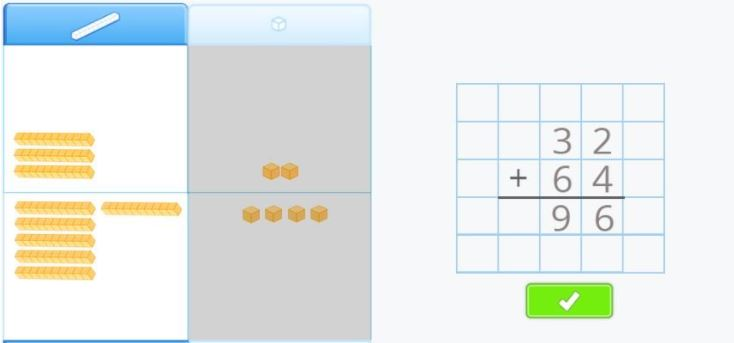### Video to Learn to Add Without Carrying

If you would like, take a look at the following video to see more examples of addition without carrying.

This is a video of one of our interactive tutorials, and although it is not interactive you have the advantage of watching it as many times as you need to and can share it with your friends. If you would like to access our interactive tutorials, register with Smartick! The online method which helps children ages 4 to 14 learn and practice math.

### How to Solve Addition With Carrying

In this case, when you add the numbers in a column and get a two-digit number, you will carry the tens and add it to the next column.

Let’s take a look at an example. Amy and Max are playing a game and pretending to shop. Amy wants to buy a bottle of water that costs 27 cents and a package of juice boxes that costs 45 cents. We need to add 27 + 45.

First, we put 45 on top of 27 and line up the columns, units with units and tens with tens.

We add the units first, 5 + 7 = 12. We put the 2 below the units column and carry the 1 to the tens column and add it to the numbers there.

1 + 4 + 2 = 7, so we put the 7 below the tens column.

The sum is 72.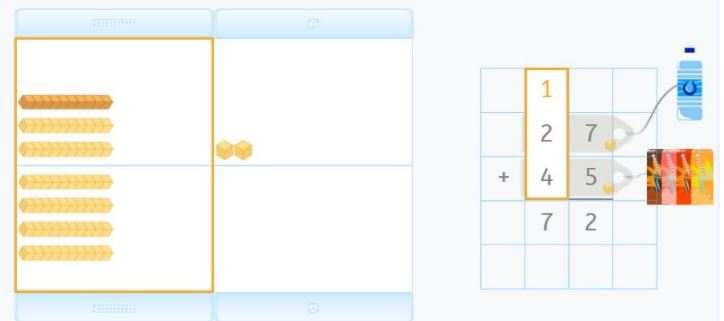### Video to Learn to Add With Carrying

Once again, we invite you to take a look at the following video to see more examples of addition with carrying.

## Exercises

### Exercise 1

In this activity, you need to select which of the vertical addition problems is written correctly. Can you find the right answer?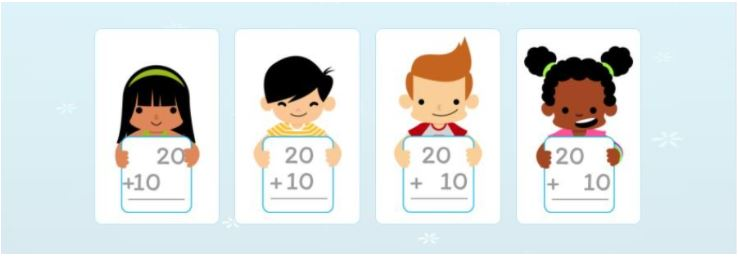Result: It is the second option.

### Exercise 2

In this activity, we are using a 100 Square to solve the addition problem. In this case, from the number 2, you can add ten units one by one or a ten, which would be the equivalent of jumping the row underneath to find the sum of the addition problem which is 12.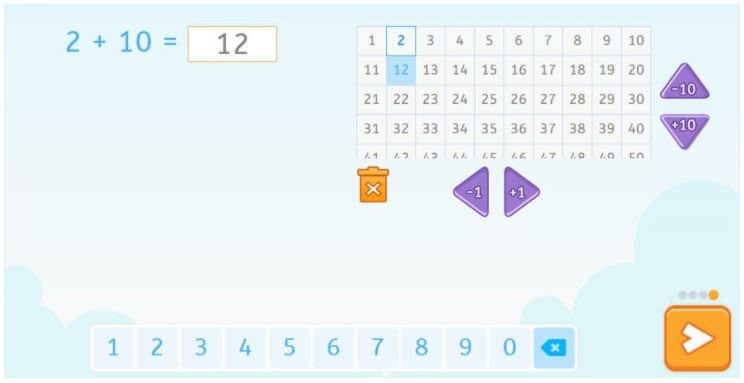### Exercise 3

We’re using the 100 Square in this activity as well and if you look closely, in this case, we are adding a ten and subtracting a unit. This way, the operation 2 + 9, is done by adding 2 + 10, which is 12, and subtracting 1, to give us the result, 11. This exercise, and material, helps to develop strategies like mental calculation.